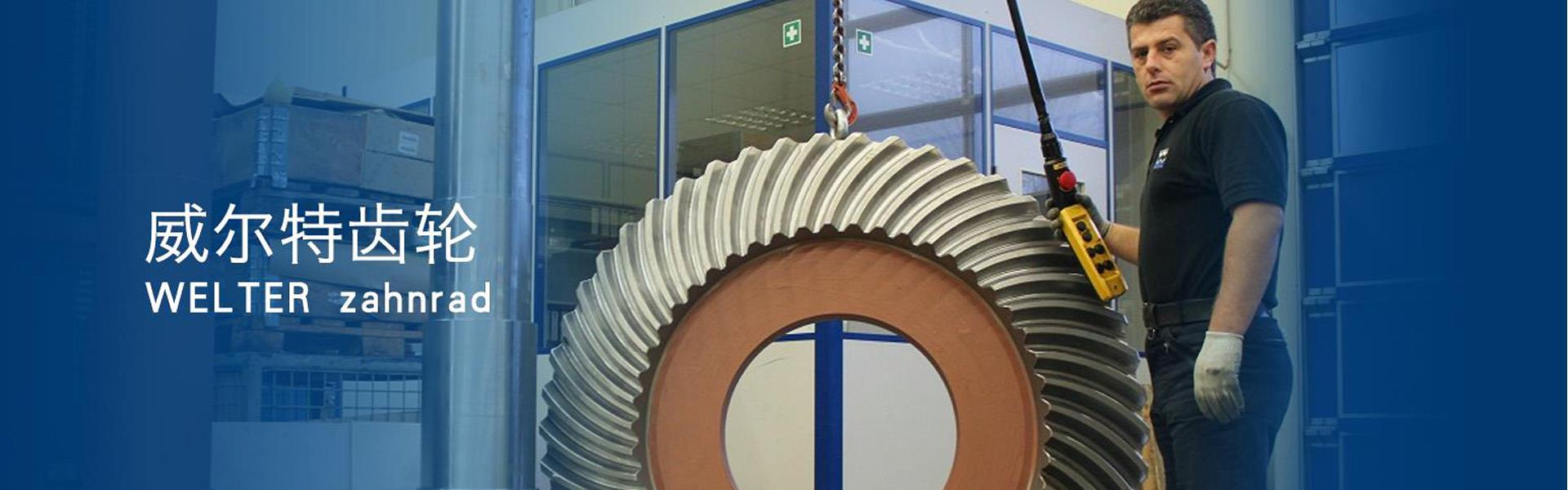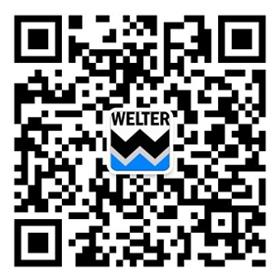Welcome to the official website of Wilt Gear (Nanjing) Co., Ltd.! Customers' peace of mind and satisfaction with each product is our lifelong pursuit. Use our efforts to solve your troubles!

China Tel
18761828866The effect of worm gear drive combination
-2019-07-09 09:05:18-

based onTurbine shaftThe failure characteristics of the transmission, when selecting the combination of worm gears and worms, not only requires sufficient strength, but also has outstanding anti-friction, wear-resistant and anti-glue ability. Practice has shown that the more ambitious material for worm gears is: bronze worm gear ring matching hardened and ground steel worm gears.

For high-speed and heavy-duty transmission, low-carbon alloy steel is commonly used for worm gears after carburizing, and then surface quenching to make the hardness 56 ~ 62HRC, and then grinding. For medium-speed and medium-load transmission, worm gears and worms are commonly used in 45 steel, 40Cr, 35SiMn, etc., and the surface is high-frequency quenched to achieve a hardness of 45-55HRC, and then ground. For general worm gears, carbon steels such as 45 and 40 can be quenched and tempered.

The axial tooth pitch pX of the worm gear should be equal to the circumferential pitch of the end face pt of the worm gear, so the axial modulus of the worm gear should be equal to the end face modulus of the worm wheel. The axial pressure angle of the worm gear should be equal to the end pressure angle of the worm wheel, indicated by α, the general standard pressure angle α=20°.

Worm gears are equivalent to spirals, and their spirals are also divided into left-handed and right-handed, single-end and multi-end. Generally, the number of heads of worm gear Z1=1～4, the more the number of heads, the higher the efficiency; but too many heads, such as Z1>4, the indexing error will increase, and it is not easy to process. The number of teeth of the worm gear Z2=iZ1, i is the transmission ratio of the worm gear drive, i=n1/n2=Z2/Z1. Regarding the general power transmission worm gear drive, Z2=27～80. When Z2< 27,="" the="" worm="" gear="" teeth="" are="" prone="" to="" undercutting;="" and="" when="" z2="" is="" too="" large,="" the="" bending="" strength="" of="" the="" worm="" gear="" teeth="" may="" be="" weak.="" use="" d1="" to="" indicate="" the="" index="" circle="" diameter="" of="" the="" worm="" wheel="" and="" worm,="" then="" the="" helix="" angle="" λ="" on="" the="" index="" cylinder="" of="" the="" worm="" wheel="" and="" worm="" can="" be="" obtained="" as="" follows.="" in="" the="" above="" formula,="" q="Z1/tgλ" can="" be="" used="" to="" obtain="" the="" index="" circle="" diameter="" of="" the="" worm="" wheel="" and="" worm.="" is="" d1="qm." in="" the="" formula,="" q="" is="" called="" the="" worm="" gear="" characteristic="" coefficient.="" in="" order="" to="" constrain="" the="" number="" of="" hobs,="" the="" q="" value="" adjusted="" to="" each="" modulus="" is="" regulated="" in="" the="" specification.="" generally="" q="6～17." the="" worm="" wheel="" index="" circle="" diameter="" d2="">

### 相关行业知识威尔特微信公众号

Address：288 Yuxiu Road, Honglan Industrial Park, Lishui District, Nanjing City, Jiangsu Province